4714.0 - National Aboriginal and Torres Strait Islander Social Survey, 2008
Previous ISSUE Released at 11:30 AM (CANBERRA TIME) 30/10/2009
 Page tools: .mffeedback,#pjs { display :none; } Enable Javascript to Print PagesPrint AllEmail NotificationRSSSearch this Product Explanatory Notes Glossary Abbreviations Comparison to other ABS data sources (Appendix) Quality Declaration Data Cubes (I-Note) Technical Note (Technical Note) TECHNICAL NOTE Estimation procedures 1 Estimates from the survey were derived using a complex estimation procedure which ensures that survey estimates conform to independent population estimates by state, part of state, remoteness area,Torres Strait Islander status, Torres Strait Islander region, age and sex. Reliability of estimates 2 Two types of error are possible in an estimate based on a sample survey: sampling error and non-sampling error. The sampling error is a measure of the variability that occurs by chance because a sample, rather than the entire population, is surveyed. Since the estimates in this publication are based on information obtained from a sample of Indigenous persons they are subject to sampling variability; that is they may differ from the figures that would have been produced if all Indigenous persons had been included in the survey. One measure of the likely difference is given by the standard error (SE). There are about two chances in three that a sample estimate will differ by less than one SE from the figure that would have been obtained if all Indigenous persons had been included, and about 19 chances in 20 that the difference will be less than two SEs. 3 Another measure of the likely difference is the relative standard error (RSE), which is obtained by expressing the SE as a percentage of the estimate. The RSE is a useful measure in that it provides an immediate indication of the percentage errors likely to have occurred due to sampling, and thus avoids the need to refer also to the size of the estimate.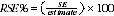4 RSEs for all estimates in this publication are available free-of-charge on the ABS website, released in spreadsheet format as an attachment to this publication, National Aboriginal and Torres Strait Islander Social Survey (cat. no. 4714.0). 5 The smaller the estimate the higher is the RSE. Very small estimates are subject to such high SEs (relative to the size of the estimate) as to detract seriously from their value for most reasonable uses. In the tables in this publication, only estimates with RSEs less than 25% are considered sufficiently reliable for most purposes. However, estimates with larger RSEs, between 25% and less than 50% have been included and are preceded by an asterisk (eg *3.4) to indicate they are subject to high SEs and should be used with caution. Estimates with RSEs of 50% or more are preceded with a double asterisk (eg **0.6). Such estimates are considered unreliable for most purposes. Standard errors of proportions and percentages 6 Proportions and percentages formed from the ratio of two estimates are also subject to sampling errors. The size of the error depends on the accuracy of both the numerator and the denominator. For proportions where the denominator is an estimate of the number of persons in a group and the numerator is the number of persons in a sub-group of the denominator group, the formula to approximate the RSE is given by: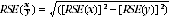7 From the above formula, the RSE of the estimated proportion or percentage will be lower than the RSE of the estimate of the numerator. Thus an approximation for SEs of proportions or percentages may be derived by neglecting the RSE of the denominator, ie by obtaining the RSE of the number of persons corresponding to the numerator of the proportion or percentage and then applying this figure to the estimated proportion or percentage. Comparison of estimates 8 Published estimates may also be used to calculate the difference between two survey estimates. Such an estimate is subject to sampling error. The sampling error of the difference between two estimates depends on their SEs and the relationship (correlation) between them. An approximate SE of the difference between two estimates (x-y) may be calculated by the following formula: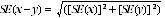9 While the above formula will be exact only for differences between separate and uncorrelated (unrelated) characteristics of sub-populations, it is expected that it will provide a reasonable approximation for all differences likely to be of interest in this publication. Significance testing 10 For comparing estimates between surveys or between populations within a survey it is useful to determine whether apparent differences are 'real' differences between the corresponding population characteristics or simply the product of differences between the survey samples. One way to examine this is to determine whether the difference between the estimates is statistically significant. This is done by calculating the standard error of the difference between two estimates (x and y) and using that to calculate the test statistic using the formula below: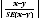11 The imprecision due to sampling variability, which is measured by the SE, should not be confused with inaccuracies that may occur because of imperfections in reporting by respondents and recording by interviewers, and errors made in coding and processing data. Inaccuracies of this kind are referred to as non-sampling error, and they occur in any enumeration, whether it be a full count or sample. Every effort is made to reduce non-sampling error to a minimum by careful design of questionnaires, intensive training and supervision of interviewers, and efficient operating procedures. Age standardisation 12 Age standardisation techniques have been used in this publication to remove the effect of the differing age structures in the Indigenous and non-Indigenous populations. The age structure of the Indigenous population is considerably younger than that of the non-Indigenous population, and age is strongly related to many health measures. Therefore, estimates of prevalence which do not take account of age may be misleading. The age standardised estimates of prevalence are those rates that 'would have occurred' should the Indigenous and non-Indigenous populations both have the standard age composition. 13 For this publication the direct age standardisation method was used. The standard population used was the total estimated resident population of Australia as at 30 June 2001. Estimates of age standardised rates were calculated using the following formula: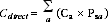14 Where Cdirect = the age standardised rate for the population of interest, a = the age categories that have been used in the age standardisation, Ca = the estimated rate for the population being standardised in age category a, and Psa = the proportion of the standard population in age category a. The age categories used in the standardisation for this publication are 15-24 years, 25-34 years, 35-44 years, 45-54 years and then 55 years and over. 15 Age standardisation may not be appropriate for particular variables, even though the populations to compare have different age distributions and the variables in question are related to age. It is also necessary to check that the relationship between the variable of interest and age is broadly consistent across the populations. If the rates vary differently with age in the two populations then there is evidence of an interaction between age and population, and as a consequence age standardised comparisons are not valid. Document Selection These documents will be presented in a new window.